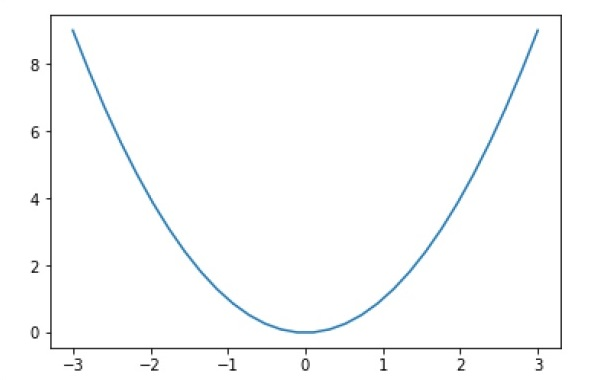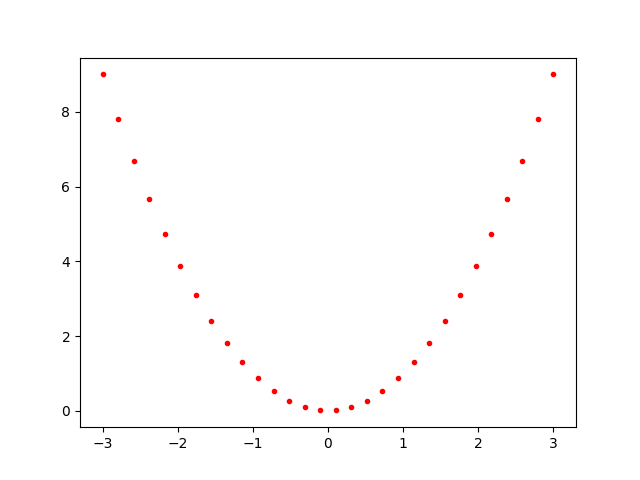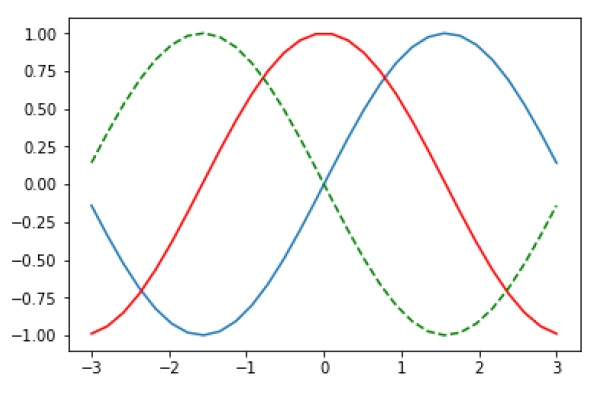# Matplotlib - PyLab module

PyLab is a procedural interface to the Matplotlib object-oriented plotting library. Matplotlib is the whole package; matplotlib.pyplot is a module in Matplotlib; and PyLab is a module that gets installed alongside Matplotlib.

PyLab is a convenience module that bulk imports matplotlib.pyplot (for plotting) and NumPy (for Mathematics and working with arrays) in a single name space. Although many examples use PyLab, it is no longer recommended.

## Basic Plotting

Plotting curves is done with the plot command. It takes a pair of same-length arrays (or sequences) −

```from numpy import *
from pylab import *
x = linspace(-3, 3, 30)
y = x**2
plot(x, y)
show()
```

The above line of code generates the following output −To plot symbols rather than lines, provide an additional string argument.

 symbols - , –, -., , . , , , o , ^ , v , < , > , s , + , x , D , d , 1 , 2 , 3 , 4 , h , H , p , | , _ colors b, g, r, c, m, y, k, w

Now, consider executing the following code −

```from pylab import *
x = linspace(-3, 3, 30)
y = x**2
plot(x, y, 'r.')
show()
```

It plots the red dots as shown below −Plots can be overlaid. Just use the multiple plot commands. Use clf() to clear the plot.

```from pylab import *
plot(x, sin(x))
plot(x, cos(x), 'r-')
plot(x, -sin(x), 'g--')
show()
```

The above line of code generates the following output −